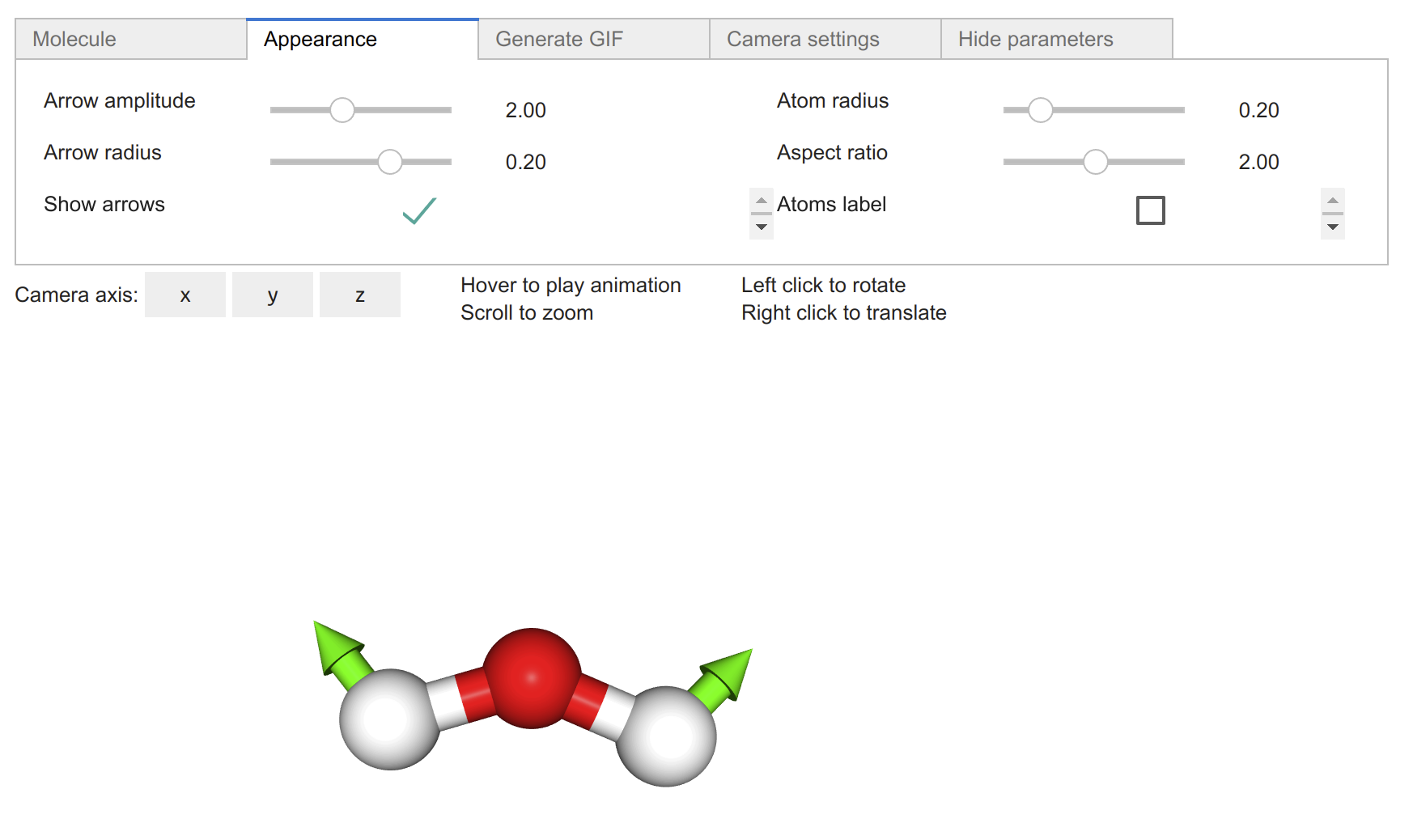# Molecular and Lattice Vibration#

## 1. Introduction to 1D Phonons#

This notebook introduces the concept of lattice vibration and their corresponding quanta of vibrational energy, the phonons. Phonon are at the heart of thermal properties of crystals such as heat capacity, thermal conduction, etc. The concept of dispersion relation, Brillouin zone and effect of diatomic lattice are discussed in duo with the interactive visualizer.## 2. 2D Phonons Dispersion#

This notebook extends the notion of phonons to the 2D case. In this notebook, the link between the reciprocal space and the band dispersion plot along certain path is made explicit. The wave can now be transversal as opposed to longitudinal only in the 1D phonon case. A simple example, the square lattice, and a more complex one, the honeycomb lattice, are presented.## 3. Introduction to Molecular Vibrations#

With this notebook, the concept of molecular vibration is explored and visualized in an interactive fashion.